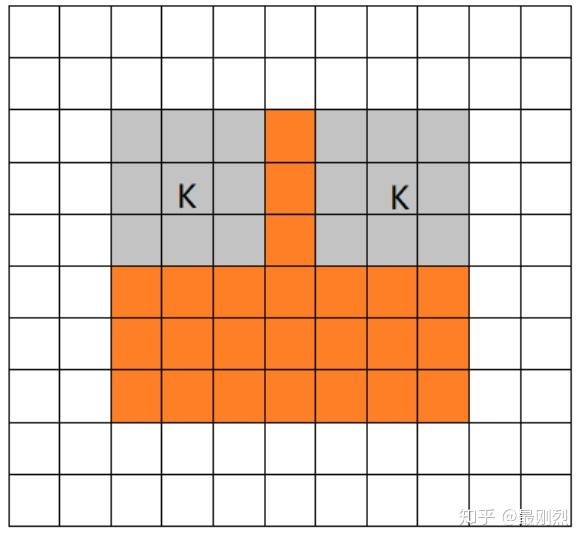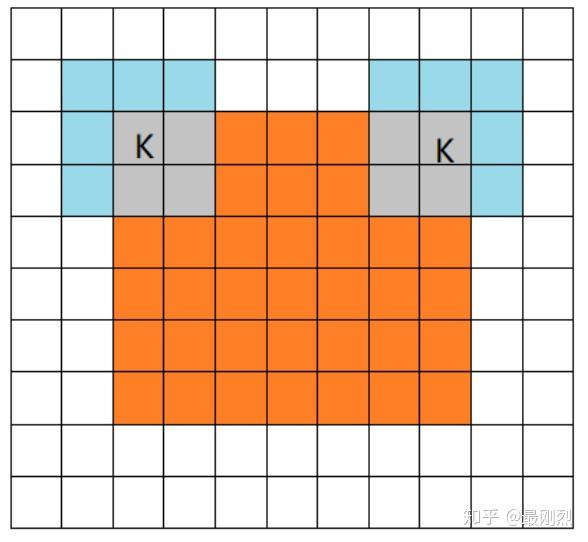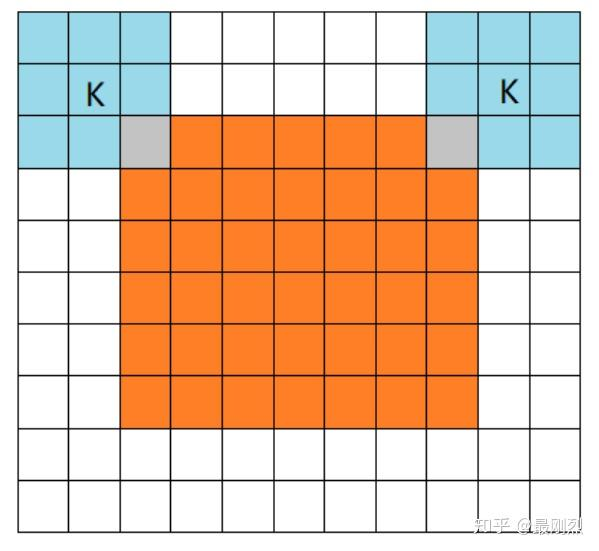# 英语中的有趣现象

### 有哪些英语单词倒过来是另一个单词？

DictionaryLookup[x__ /; DictionaryWordQ@StringReverse@x]

### 有哪些英语单词是回文的？

DictionaryLookup[x__ /; x === StringReverse@x]

DictionaryLookup[x__ /; PalindromeQ@x]

### 哪两个字母不可能连在一起？

https://www.zhihu.com/question/25908268

### 英文各字母使用频率？

https://www.zhihu.com/question/23111432

### 英语单词 26 个首字母出现概率各是多少？

https://www.zhihu.com/question/30077599

## Valid Mode• 输入图片大小: W*W

• Filter大小:

• 步长: S

N = (W- F+ 2P)/S+1

$$n_{\text {output}}=\left[\frac{n_{\text {input}}-f+1}{s}\right]$$

conv2d的VALID方式不会在原有输入的基础上添加新的像素（假定我们的输入是图片数据，因为只有图片才有像素），输出矩阵的大小直接按照公式计算即可。

## Same Mode$$n_{\text {output}}=\left[\frac{n_{\text {input}}}{s}\right]$$

pad_needed_height = (new_height [Dash] 1) * S + F - W

pad_needed_width = (new_width [Dash] 1) * S + F - W

## Full Mode# CV 模型的标准化

## NCHW or NHWC

NCHW 下取第一张图片就是 img, NHWC 则要写 img[:,:,:,1], 何苦啊这是...

## 左下系 or 左上系

(所以为什么不规定为矩阵从下开始数...

### 解决方案

\min{}
} 和 {y_
\max{}
} 对调一下.

# Windows 全深度学习环境安装

conda create -n deeplearning python=3.7
conda activate deeplearning

conda config --add channels https://mirrors.tuna.tsinghua.edu.cn/anaconda/pkgs/main/
conda config --set show_channel_urls yes
conda config --show channels

conda config --remove channels https://mirrors.tuna.tsinghua.edu.cn/anaconda/pkgs/free/

conda install tensorflow keras caffe-cpu pytorch-cpu -y
pip install mxnet

# 寻找数列万能公式

### 初等函数

$$p_n=1+\sum_{m=1}^{2^n}\left\lfloor \sqrt[n]{\left\lfloor n\biggl/\sum_{k=1}^m {\left\lfloor \cos^{2} \lfloor \pi\dfrac{(k-1)!+1}{k} \rfloor \right\rfloor} \right\rfloor} \right\rfloor$$

1. 只用加减乘除的公式叫做 算数表达式(Arithmetic expressions).

2. 加入幂, 开根之后叫做 代数表达式(Algebraic expressions).

3. 允许使用初等函数的公式叫做 封闭形式(Closed-form expressions), 也可以叫初等表达式.

4. 允许使用级数算符的公式叫 解析形式(Analytic expressions), 其中级数要求收敛, 不能是形式幂级数.

5. 剩下的统称为数学定义式(Mathematical expressions), 允许使用包括极限、微分与积分算符在内的一切数学符号, 级数可以是单点收敛的形式幂级数, 甚至式子不必用等式定义, 直接说是某个方程的解.

### 分段函数

![](https://i.loli.net/2018/03/24/5ab63a46384a5.gif)

### 插值函数

$n$ 个点最多能确定了一个 $n-1$ 次的多项式.

$$\begin{cases} y_1 = a_0 + a_1x_1 + a_2x_1^2+\cdots+a_nx_1^n\\ y_2 = a_0 + a_1x_2 + a_2x_2^2+\cdots+a_nx_2^n\\ y_3 = a_0 + a_1x_3 + a_2x_3^2+\cdots+a_nx_3^n \\ \cdots\cdots\cdots\cdots\cdots\cdots\cdots\cdots\cdots\cdots\cdots \\ y_n = a_0 + a_1x_n + a_2x_n^2+\cdots+a_nx_n^n \end{cases}$$

$$\begin{pmatrix} y_1 \\ y_2 \\ y_3 \\ \vdots\\y_n \end{pmatrix} = \begin{pmatrix} 1 & x_1 & x_1^2 &\cdots&x_1^n\\ 1 & x_2 & x_2^2 &\cdots&x_2^n\\ 1 & x_3 & x_3^2 &\cdots&x_3^n\\ \vdots&\vdots&\vdots&\ddots&\vdots&\\ 1 & x_n & x_n^2 &\cdots&x_n^n\\ \end{pmatrix} \begin{pmatrix} a_0 \\ a_1 \\ a_2 \\ \vdots\\a_n \end{pmatrix}$$

$$\vec{y}=X\vec{a}$$

$$P(x):=\sum_{j=0}^{k}y_{j}\prod _{0\leq m\leq k}^{m\neq j}{\frac {x-x_{m}}{x_{j}-x_{m}}}$$

![](https://i.loli.net/2018/03/24/5ab63a461dd88.png)

### 生成函数

$$\frac{B_n}{n!}=[z^n]\left(\frac{z}{e^z-1}\right)$$

### 后记

data=BlockRandom[SeedRandom;Table[Exp[-3 x/(2+ x+x^2)]+RandomReal[{-.5,.5}],
{x,-10,10}]
];
p[i_]:=ListLinePlot[Labeled[data,Text@Style[ToString@i<>"次插值",15],{Scaled[0.35],Above}],AspectRatio->1/3,
InterpolationOrder->i,PlotRange->{0,6},
Mesh->Full,MeshStyle->Blue,ImageSize->Large
];
Export["万能公式_1.gif",p/@Range[0,10],
"AnimationRepetitions"->Infinity,
"DisplayDurations"->1
]

# 21道犹太棺材题(上)

### Question 1

$\displaystyle{x(8\sqrt{1-x}+\sqrt{1+x})\leq11\sqrt{1+x}-16\sqrt{1-x}}$

### Question 5

$\displaystyle{2 \sqrt{2 y-1} = y^3 + 1}$

# 计算

## 烷基自由基计数

### Planarisomers

\cong{}
\mathbb{\Z{}}
_3

$$\mathbb{Z}(\mathcal{S}_3)=\frac 16 (2s_3+3s_2s_1+s_1^3)$$

\begin{aligned} A(x)&=1+x\mathbb{Z}[\mathcal{S}_3,A(x)]\\ &=1+\dfrac{x}{6}[A^3(x)+3A(x)A(x^2)+2A(x^3)] \end{aligned}

A[z_]:=Evaluate@Normal@Fold[
Series[1+z/6(#^3+3# ComposeSeries[#,z^2+O[z]^#2]+2 ComposeSeries[#,z^3+O[z]^#2]),{z,0,#2}]&,
1+O[z],Range@Floor@n
];

### Stereoisomers

$$\mathbb{Z}(\mathcal{C}_3)=\frac13(s_1^3+2 s_3)$$

A[z_]:=Evaluate@Normal@Fold[
Series[1+z/3(#^3+2 ComposeSeries[#,z^3+O[z]^#2]),{z,0,#2}]&,
1+O[z],Range@Floor[n/2]
];

## 烷烃计数

$$p^*-q^*+s=1$$

let Alkane Count,$\mathtt{Ac}$

\mathtt{\Ac{}}

(z)=P(z)-Q(z)+S(z)

### Planarisomers

\begin{aligned} P(x)&=x\mathbb{Z}[\mathcal{S}_4,A(x)]\\ &=\frac{1}{24}x(A^4(x)+6A^2(x)A(x^2)+3A^2(x^2)+8A(x)A(x^3)+6A(x^4))\\ Q(x)&=\mathbb{Z}[\mathcal{S}_2,A(x)-1]\\ &=\frac 12((A(x)-1)^2+A(x^2)-1)\\ S(x)&=A(z^2)-1 \end{aligned}

AlkaneSeries[n_Integer]:=Block[
{A,P,Q,S,G},
A[z_]:=Evaluate@Normal@Fold[
Series[1+z/6(#^3+3# ComposeSeries[#,z^2+O[z]^#2]+2 ComposeSeries[#,z^3+O[z]^#2]),{z,0,#2}]&,
1+O[z],Range@Floor[n/2]
];
P[z_]=z CycleIndexPolynomial[SymmetricGroup,Array[A[z^#]&,4]];
Q[z_]=CycleIndexPolynomial[SymmetricGroup,Array[A[z^#]-1&,2]];
S[z_]=A[z^2];
Series[P[z]-Q[z]+S[z]-1,{z,0,n}]
];

### Stereoisomers

$$\mathbb{Z}(\mathcal{A}_4)=\frac{1}{12}(s_1^4+8 s_3 s_1+3 s_2^2)$$

$$Q(x)=\mathbb{Z}[\mathcal{A}_4,A(x)]=\frac{1}{12}\left(8 A\left(z^3\right) A(z)+3 A\left(z^2\right)^2+A(z)^4\right)$$

AlkaneSeries[n_Integer]:=Block[
{A,P,Q,S,G},
A[z_]:=Evaluate@Normal@Fold[
Series[1+z/3(#^3+2 ComposeSeries[#,z^3+O[z]^#2]),{z,0,#2}]&,
1+O[z],Range@Floor[n/2]
];
P[z_]=z CycleIndexPolynomial[AlternatingGroup,Array[A[z^#]&,4]];
Q[z_]=CycleIndexPolynomial[SymmetricGroup,Array[A[z^#]-1&,2]];
S[z_]=A[z^2];
Series[P[z]-Q[z]+S[z]-1,{z,0,n}]
];

## 苯环

pg = GroupDirectProduct[AlternatingGroup@5, CyclicGroup@2];
A[z_, i_] := Evaluate[#^i & /@ (1 + a + b + c + d)]
CycleIndexPolynomial[pg, Array[A[z, #] &, 60]];
Coefficient[%, a b c d]

# 附录

S,C,A,D

### 群直积与积群

GroupDirectProduct[g1_,g2_]:=With[
{order1=GroupOrder@g1,order2=GroupOrder@g2,r,pd},
pd=Outer[PermutationProduct,GroupElements[g1],GroupElements[g2]/.r];
PermutationGroup@Flatten@pd
]

GroupDirectProduct[AlternatingGroup@5,CyclicGroup@2]
FiniteGroupData[{"DirectProduct",
{
{"AlternatingGroup",5},
{"CyclicGroup",2}
}
},"PermutationGroupRepresentation"]